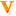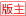·社区中心 社区公告| 政策解答| 学员心声 ·考试专区 中级综合区| 中级会计实务| 中级经济法| 中级财务管理| 初级职称 注会综合区| 会计| 审计| 税法| 经济法| 财管| 风险管理| 综合阶段 税务师| 资产评估师| 高级会计师| 审计师| 经济师 会计学历考试| 国际证书考试| 银行/证券/统计/期货从业 ·互动交流 正保财务软件| 名师专区| 嘉宾访谈| 休闲娱乐| 招聘求职| 会计新人秀 生活与健康| 大学来啦 ·版务专区 南京继续教育| 保定继续教育

# 帖子主题： [每日一测] 【最后冲刺】年金终值与现值（计算分析题）发表于：2017-10-12 10:48:12

 三千筱夜5987主题帖 11042问答数 论坛等级: 论坛元老 回帖：41831 经验：161003 鲜花：260 金币：66380 离线 用户信息 加为好友0点击：949 回复：5    楼主 　　【例题 计算分析题】小Z2015年1月1日存入银行100万元，年利率为8%。分别回答下列各不相关的问题：　　(1)每季度复利一次，则2018年1月1日存款账户余额为多少?　　(2)假设分别在2015年、2016年、2017年、2018年的1月1日存入银行100万元，年利率依然为8%，则2018年1月1日的账户余额为多少?　　(3)假设分别在2015年、2016年、2017年、2018年的1月1日存入银行100万元，年利率依然为8%，则2018年12月31日的账户余额为多少?　　(4)假定从2015年1月1日开始分3期每年年初存入银行相等的金额，为在2018年的1月1日达到第一问所得到的账户余额，每期应存入多少金额?　　　　(1)F=100×(F/P，8%/4,4×3)=100×(F/P，2%,12)=100×1.2682=126.82(万元)　　(2)F=100×(F/A，8%，4)=100×4.5061=450.61(万元)　　(3)F=100×(F/A，8%，4)×(1+8%)=100×4.5061×1.08=486.66(万元)　　(4)A=126.82/(F/A，8%，3)=126.82/3.2464=39.06(万元)。 悬赏金额: 30 金币 状态： 已解决 最佳答案:(1)F=100×(F/P，8%/4,4×3)=100×(F/P，2%,12)=100×1.2682=126.82(万元)　　(2)F=100×(F/A，8%，4)=100×4.5061=450.61(万元)　　(3)F=100×(F/A，8%，4)×(1+8%)=100×4.5061×1.08=486.66(万元)　　(4)A=126.82/(F/A，8%，3)=126.82/3.2464=39.06(万元)。 收藏 求助 送鲜花 举报 相关热帖 楼主其他文章
 三千筱夜5987主题帖 11042问答数 论坛等级: 论坛元老 回帖：41831 经验：161003 鲜花：260 金币：66380 离线 用户信息01楼   发表于：2017-10-12 10:48:37 只看该作者@eddie1121 @1604 @yangshuai67 @少客 @淡忘回忆 @05869 @李喆不是李哲 @奋斗的小鸟 @凤九 @FNC @冰冰 @19851012770520 @欣洋宇苑 @_四叶草_ @805368400 @蓝蓝的海 @红桃Q @天佑 @echoho @蛋炒饭的脑残粉
 11525主题帖 3560问答数 论坛等级: 论坛元老 回帖：32944 经验：184489 鲜花：1044 金币：8784 离线 用户信息02楼   发表于：2017-10-12 15:09:00 只看该作者(1)F=100×(F/P，8%/4,4×3)=100×(F/P，2%,12)=100×1.2682=126.82(万元)　　(2)F=100×(F/A，8%，4)=100×4.5061=450.61(万元)　　(3)F=100×(F/A，8%，4)×(1+8%)=100×4.5061×1.08=486.66(万元)　　(4)A=126.82/(F/A，8%，3)=126.82/3.2464=39.06(万元)。 三千筱夜 于 2017-10-13 14:00:28 回复： 　　(1)F=100×(F/P，8%/4,4×3)=100×(F/P，2%,12)=100×1.2682=126.82(万元)　　(2)F=100×(F/A，8%，4)=100×4.5061=450.61(万元)　　(3)F=100×(F/A，8%，4)×(1+8%)=100×4.5061×1.08=486.66(万元)　　(4)A=126.82/(F/A，8%，3)=126.82/3.2464=39.06(万元)。 努力的终点是无能为力，拼搏的终点是感动自己。
 zgaominmin183主题帖 5064问答数 论坛等级: 终极会员 回帖：14133 经验：30256 鲜花：572 金币：8771 离线 用户信息03楼   发表于：2017-10-13 08:52:38 只看该作者 学习 三千筱夜 于 2017-10-13 14:00:51 回复： (1)F=100×(F/P，8%/4,4×3)=100×(F/P，2%,12)=100×1.2682=126.82(万元)　　(2)F=100×(F/A，8%，4)=100×4.5061=450.61(万元)　　(3)F=100×(F/A，8%，4)×(1+8%)=100×4.5061×1.08=486.66(万元)　　(4)A=126.82/(F/A，8%，3)=126.82/3.2464=39.06(万元)。 想要到达明天，现在就要启程。

# 【最后冲刺】年金终值与现值（计算分析题）您尚未登录,发表回复前请先登录，或者注册
 Ctrl+Enter直接发表